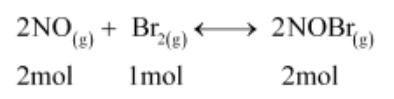# Nitric oxide reacts with Br2 and gives nitrosyl bromide as per reaction given below:

Question:

Nitric oxide reacts with Br2 and gives nitrosyl bromide as per reaction given below:

$2 \mathrm{NO}(\mathrm{g})+\mathrm{Br}_{2}(\mathrm{~g}) \longleftrightarrow 2 \mathrm{NOBr}(\mathrm{g})$

When $0.087 \mathrm{~mol}$ of $\mathrm{NO}$ and $0.0437 \mathrm{~mol}$ of $\mathrm{Br}_{2}$ are mixed in a closed container at constant temperature, $0.0518 \mathrm{~mol}$ of $\mathrm{NOBr}$ is obtained at equilibrium. Calculate equilibrium amount of $\mathrm{NO}$ and $\mathrm{Br}_{2}$.

Solution:

The given reaction is:Now, 2 mol of NOBr are formed from 2 mol of NO. Therefore, 0.0518 mol of NOBr are formed from 0.0518 mol of NO.

Again, 2 mol of NOBr are formed from 1 mol of Br.

Therefore, $0.0518 \mathrm{~mol}$ of $\mathrm{NOBr}$ are formed from $\frac{0.0518}{2} \mathrm{~mol}$ of $\mathrm{Br}$, or

0.0259 mol of NO.

The amount of NO and Br present initially is as follows:

[NO] = 0.087 mol [Br2] = 0.0437 mol

Therefore, the amount of NO present at equilibrium is:

[NO] = 0.087 – 0.0518

= 0.0352 mol

And, the amount of Br present at equilibrium is:

[Br2] = 0.0437 – 0.0259

= 0.0178 mol